# GRE Subject Test: Math : Trigonometric Functions

## Example Questions

### Evaluate: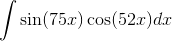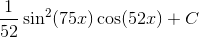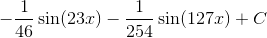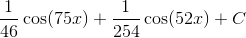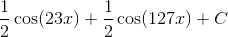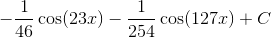Explanation:

Evaluating this integral requires use of the "Product to Sum Formulas of Trigonometry":

For: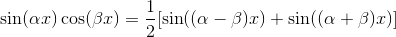So for our given integral, we can rewrite like so: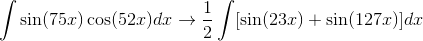This can be rewritten as two separate integrals and solved using a simple substitution.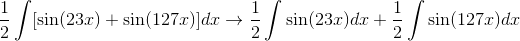Solving each integral individually, we have: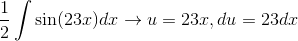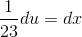Substituting this into the integral results in: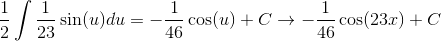The other integral is solved the same way: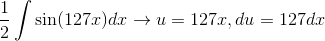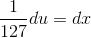Substituting this into the integral results in: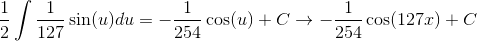Now combining these two statements together results in one of the answer choices:### Evaluate: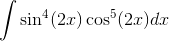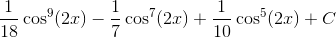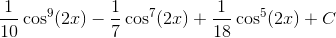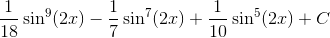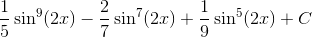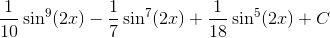Explanation:

This integral can be easily evaluated by following the rules outlined for integrating powers of sine and cosine.

But first a substitution needs to be made: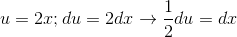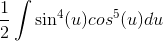Now that we've made this substitution, we will use the rules outlined for integrating powers of sine and cosine:

In General: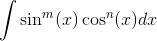1. If "m" is odd, then we make the substitution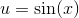, and we use the identity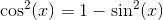.

2. If "n" is odd, then we make the substitution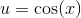, and we use the identity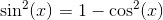.

For our given problem statement we will use the first rule, and alter the integral like so: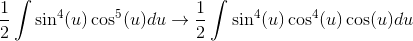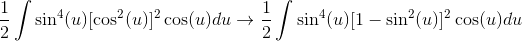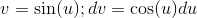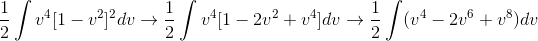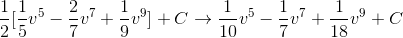Now we need to substitute back into v: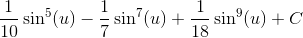Now we need to substitute back into u, and rearrange to make it look like one of the answer choices:### Example Question #3 : Trigonometric Functions

Find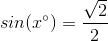Explanation:

Step 1: Draw a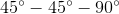triangle..

The short sides have a length ofand the hypotenuse has a length of.

Step 2: Find Sin (Angle A):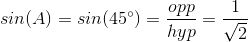Step 3: Rationalize the root at the bottom: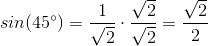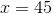### Example Question #71 : Functions And Graphs

Evaluate the derivative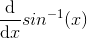1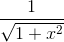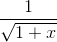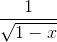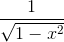Explanation:

The inverse trig functions should be memorized.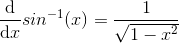The other common inverse trig functions are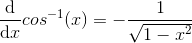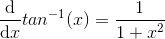### All GRE Subject Test: Math Resources